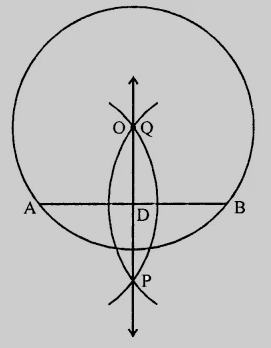# Draw a circle with centre at point $O$ and radius $5\ cm$. Draw its chord $AB$, draw the perpendicular bisector of line segment $AB$. Does it pass through the centre of the circle?

Given:

A circle with centre at point $O$ and radius $5\ cm$.

To do:

We have to draw the circle, its chord $AB$ and the perpendicular bisector of line segment $AB$.

Solution:Steps of construction:

(i) Draw a circle with centre $O$ and radius $5\ cm$.

(ii) Draw a chord $AB$ of the circle.

(iii) With centres $A$ and $B$ and radius more than half of $AB$ draw arcs intersecting each other at $P$ and $Q$.

(iv) Join $PQ$ which intersects $AB$ at $D$ and passes through $O$, the centre of the circle.

This is the required circle.

Updated on: 10-Oct-2022

66 Views